# Reddit for Fun and Profit [part 2]

In the prior post Tracking Posts on WallStreetBets - Part I, we demonstrated how relatively easy it is to extract reddit activities related to a given stock ticker - in their raw form. If you haven't already read that post, you may want to take a moment to skim that article.

In this post, we are going to take the next obvious step: aggregating the raw results into a meaningful timeseries measure of posting activity. With a few important transforms, we can generate time series and evaluate them

As always, if you'd like to replicate and experiment with the below code, you can download the source notebook for this post by right-clicking on the below button and choosing "save link as"## Setup¶

This step will only require a few simple packages which you likely already have: pandas, re, collections, and datetime (all but pandas are part of the standard python library).

We will also define a few variables. DATA_ROOT is the (relative or absolute) path to the folder that contains the data downloaded in Part I. symbols is the list of tickers for which we want to analyze. Each must have Reddit data already downloaded, of course.

In :
import os
import re
from collections import Counter
import pandas as pd
from datetime import datetime

import matplotlib.pyplot as plt
%matplotlib inline

DATA_ROOT = '../data/'
symbols = ['GME','AMC','SPCE','TSLA']


Now that the data is loaded, I'll create a super simple function to read ajsonlines file from disk into a dataframe. We can do this directly with the pandas.read_json() method. Note that lines=True must be set in order for the jsonlines file format to work. Just in case we have any dirty files - for instance if we ran the downloader function multiple times which caused duplicates - we will drop duplicate rows.

In :
def read_file(symbol):
path = os.path.join(DATA_ROOT, f'reddit/{symbol}.jl')
return df

df.columns

Out:
Index(['author', 'created_utc', 'id', 'num_comments', 'score', 'selftext',
'subreddit', 'title', 'url', 'created', 'date_utc'],
dtype='object')

That successfully reads a jsonlines file into memory as a dataframe with 11 columns.

Next, we need to do an important step to filter out false positives (i.e., posts which falsely showed up as relating to a ticker). This can happen if the ticker in question also appears in common english language. For instance, searching for Kimco Realty Corp (ticker: KIM) yields many posts about Kim Kardashian and other annoying and irrelevant subjects.

To correct this, we will make a function that uses a simple regex to find only the matches which are 2,3,or 4 character words in all caps. Not fool-proof but it will catch the vast majority of false positives.

In :
string = '''
$KIM is the ticker for Kimco Realty. \n Kim Kardashian is a heavy user of$FB and $TWTR. \n Kimchi is a delicious food. ''' exp = r'([A-Z]{2,4})' stocks = Counter() for line in string.split(' '): stocks.update(re.findall(exp, line)) stocks.most_common() s = pd.Series(stocks) s  Out: KIM 1 FB 1 TWTR 1 dtype: int64 At the same time, I'd like to measure how many total tickers were listed in a post. In my opinion, the two below examples should not be given equal weight as attention paid to $GME:

• Example 1: $GME is headed for a big week. To the moon! • Example 2: Good luck to all!$AMC $AMD$SPCE $YOLO$DOGE $GME$BTC $TSLA$CRM $ARKK! The first is clearly a post about the stock in question. The second is sort of a grab bag of tickers which does include GME. To accomplish this, we'll make the function count not only the symbol we're searching for but also all other (probable) tickers mentioned, then return the fraction of all ticker mentions which is made up of our target ticker. Example 1 would return a value of 1.0. Example 2 would return a value of 0.1 since $GME was one of ten mentioned tickers.

In :
import re
from collections import Counter

def count_tickers(string, symbol):
exp = r'([A-Z]{2,4})'
stocks = Counter()
for line in string.split(' '):
stocks.update(re.findall(exp, line))
stocks.most_common()
s = pd.Series(stocks)
try:
return s.divide(s.sum()).loc[symbol]
except:
return 0.
string = 'Good luck to all! $AMC$AMD $SPCE$YOLO $DOGE$GME $BTC$TSLA $CRM$ARKK!'
score = count_tickers(string,'GME')
print(f'String: {string}','\n',f'Score: {score}')

String: Good luck to all! $AMC$AMD $SPCE$YOLO $DOGE$GME $BTC$TSLA $CRM$ARKK!
Score: 0.1


We'll apply that function to the dataframe and place the result into a column named pct_tickers which reflects the percent of ticker mentions in the post that matched the target symbol. Since mentions could happen within either the title or body (which is called "selftext" in redditspeak), we'll concatenate those strings and apply the function to that.

This also helps us find and remove the 795 posts (about 4% of the 18K total posts) which were false positives (ie the ticker count = 0.00).

In :
symbol = 'GME'

df['pct_tickers'] = df.apply(lambda row: count_tickers(str(row['title'])+' '+str(row['selftext']), symbol), axis=1)

<ipython-input-4-434762af2c17>:10: DeprecationWarning: The default dtype for empty Series will be 'object' instead of 'float64' in a future version. Specify a dtype explicitly to silence this warning.
s = pd.Series(stocks)

In :
print(f'There were {(df.pct_tickers==0).sum()} false positives to remove...')

df = df[df.pct_tickers>0.] # remove false positives entirely

There were 836 false positives to remove...


Before moving onto analyzing the data, there are a couple more transforms to do.

1. First, we will create a new column named date which strips away the HH:MM:SS from the datetime. This is helpful so that we can aggregate all of the mentions within a given day, not a given second.
2. Next, we'll calculate the product of num_comments and pct_tickers to reflect both measures into a new metric named wtd_comments. This is the best single measure of how significant and focused the ticker's mention was in each post. A post which only mentioned the target ticker that had 100 comments would get a score of 100, while one that had 100 comments but 10 tickers would get a score of 10 (100 * 1/10) and so forth. You can make your own judgements about how to best reflect the true significance of each post but this seems like a decent first order approximation.
In :
df['date'] = pd.to_datetime(df['date_utc'].str[:10])


## Analysis¶

With all of that data prep behind us, the analysis will be dead simple. We'll start by plotting the rolling 7-day average wtd_comments for $GME like this: In : comments = df.groupby('date')['wtd_comments'].sum().rolling(7).mean().rename('reddit_volume') comments.plot(kind='area', alpha=0.33,figsize=(12,12), title = f'Seven Day rolling volume (weighted) of Reddit comments on {symbol} by week')  Out: <AxesSubplot:title={'center':'Seven Day rolling volume (weighted) of Reddit comments on GME by week'}, xlabel='date'>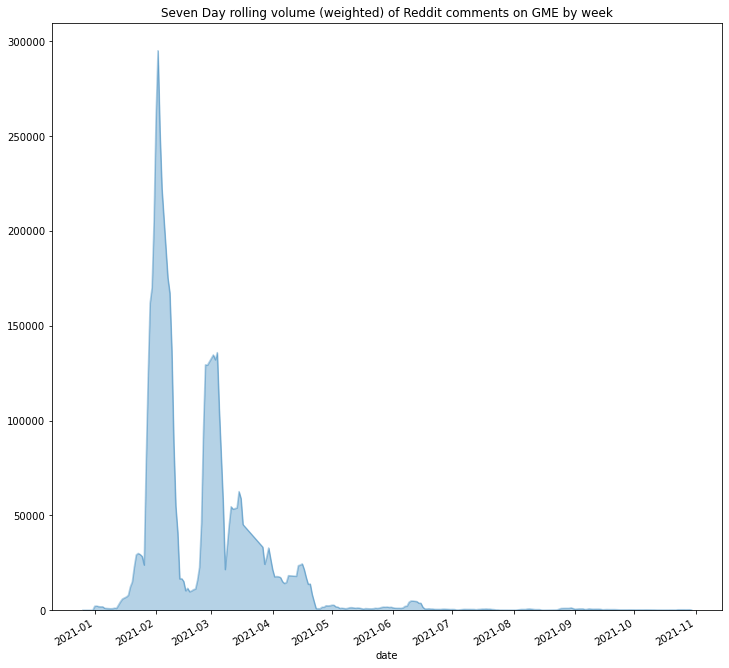Interesting, eh? The r/wallstreetbets activity tracks pretty closely to what I might have guessed. Huge spike at the end of January when "Roaring Kitty" was front-page news and$GME price was en route to the moon. I am less clear about what the late February spike was all about.

How might this be useful? Well, in an ideal world this creates a tradeable alpha signal - more comments, more money. I am more than a bit skeptical of that naive approach. A slightly more sensible application might be to predict dollar volatility with reddit comment activity. This is a much lower bar, yet still could be useful.

In :
# simple function to get dollar vol of an asset over a specified lookback window
def get_volatility(symbol, lookback=7):
path = os.path.join(DATA_ROOT,f'prices/{symbol}.csv')
volatility = prices.diff().abs().divide(prices).rolling(lookback).mean()['2021':].rename(f'{lookback}_day_volatility')
return volatility

get_volatility('GME').tail()

Out:
date
2021-10-26    0.023213
2021-10-27    0.024674
2021-10-28    0.031382
2021-10-29    0.030138
2021-11-01    0.039767
Name: 7_day_volatility, dtype: float64
In :
lookback = 7

volatility = get_volatility('GME')

title = 'Reddit Comment Activity vs. Stock Volatility: GME'
volatility.divide(volatility.max()).plot(kind='area', alpha = 0.3, legend=True, ylim=(0,None))

Out:
<AxesSubplot:title={'center':'Reddit Comment Activity vs. Stock Volatility: GME'}, xlabel='date'>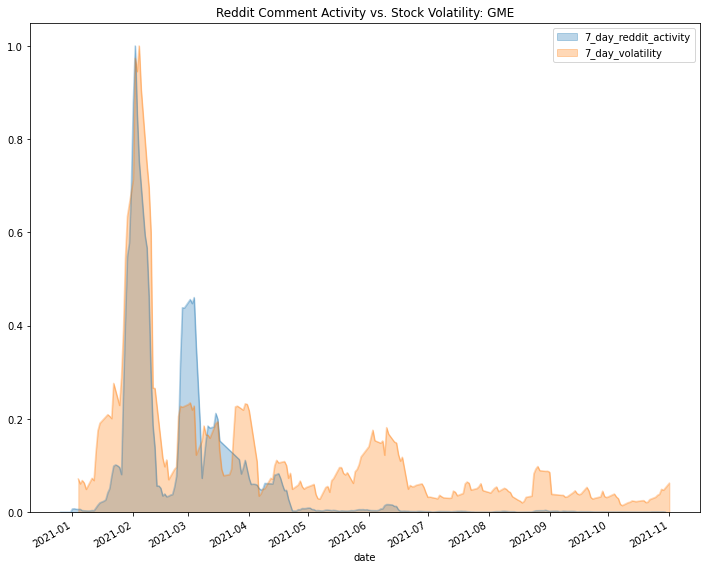This is probably as you'd expect given the headlines during the Roaring Kitty Affair. Spikes in stock price volatility (orange) were pretty tightly coupled with spikes in r/wallstreetbets comments. What is less clear is causality. Does reddit comment activity lead to price volatility or is reddit commentary the consequence of price action?

To explore this, I'll define a quick-and-dirty lead/lag correlation plot as shown below. If the red line falls to the left of the grey zero-line, that suggests possible leading relationship between comment volume and price volatility - to the right is of course the opposite. Take this with a grain of salt, but it's a first approximation.

In :
both = pd.DataFrame({'reddit':comments, 'volatility':volatility}).fillna(0)

# find lags

lag_vals = list(range(-7,8))
corrs = pd.Series()
for lag in lag_vals:
corrs.loc[lag] = corr

max_corr = corrs.max()
max_corr_lag = corrs.idxmax(max_corr)
ax = corrs.plot(title = f'Correlation at Varying Leads and Lags\n',figsize=(10,4))
ax.axvline(x=0,color='grey',alpha=0.5)
ax.axvline(x=max_corr_lag,ls='--',color='red')
ax.set_xlabel("Days Lag between comment volume and price volatility\n (-) means reddit activity LEADS volatility \n (+) means reddit activity LAGS volatility")

<ipython-input-11-b8ea46b74fec>:7: DeprecationWarning: The default dtype for empty Series will be 'object' instead of 'float64' in a future version. Specify a dtype explicitly to silence this warning.
corrs = pd.Series()

Out:
Text(0.5, 0, 'Days Lag between comment volume and price volatility\n (-) means reddit activity LEADS volatility \n (+) means reddit activity LAGS volatility')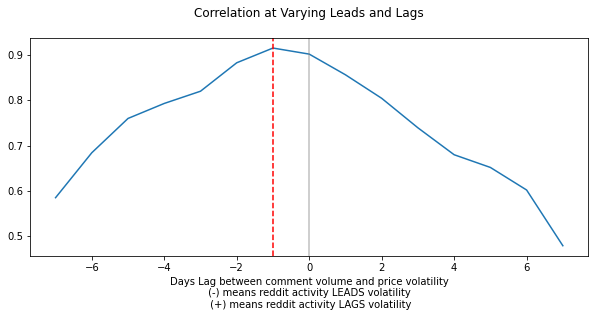Not incredibly strong, but this shows that reddit activity leads price volatility by a day or so.

Since $GME is only one of four symbols we've gotten reddit data for, we could try a few others to see if this relationship holds. Below is an all-in-one-step version of the above code to make it more repeatable across symbols In : symbol = 'TSLA' lookback = 7 # load, transform, and scale the reddit post data df = read_file(symbol) df['pct_tickers'] = df.apply(lambda row: count_tickers(str(row['title'])+' '+str(row['selftext']), symbol), axis=1) print(f'There were {(df.pct_tickers==0).sum()} false positives to remove...') df = df[df.pct_tickers>0.] # remove false positives entirely df['date'] = pd.to_datetime(df['date_utc'].str[:10]) df['wtd_comments'] = df['num_comments']*df['pct_tickers'] comments = df.groupby('date')['wtd_comments'].sum().rolling(lookback).mean().rename(f'{lookback}_day_reddit_activity') comments = comments.divide(comments.max()) # load and scale the price volatility data volatility = get_volatility(symbol) # fetch volatility = volatility.divide(volatility.max()) # normalize # Calculate the lead/lag relationship lag_vals = list(range(-7,8)) corrs = pd.Series() for lag in lag_vals: corr = volatility.shift(lag,freq='d').corr(comments) corrs.loc[lag] = corr max_corr = corrs.max() max_corr_lag = corrs.idxmax(max_corr) # Draw plots fig,axs = plt.subplots(1,2, figsize=(12,6)) # timeseries plot comments.plot(kind='area', ax=axs, alpha = 0.3, legend=True, ylim=(0,None), title=title) volatility.plot(kind='area', ax=axs, alpha = 0.3, legend=True, ylim=(0,None)) # lead/lag plot # title = f'Reddit Comment Activity vs. Stock Volatility: {symbol}' corrs.plot(title = f'Correlation at Varying Leads and Lags\n',ax=axs) axs.axvline(x=0,color='grey',alpha=0.5) axs.axvline(x=max_corr_lag,ls='--',color='red') ax.set_xlabel("Days Lag between comment volume and price volatility\n (-) means reddit activity LEADS volatility \n (+) means reddit activity LAGS volatility")  <ipython-input-4-434762af2c17>:10: DeprecationWarning: The default dtype for empty Series will be 'object' instead of 'float64' in a future version. Specify a dtype explicitly to silence this warning. s = pd.Series(stocks)  There were 74 false positives to remove...  <ipython-input-12-40ce87c792fe>:20: DeprecationWarning: The default dtype for empty Series will be 'object' instead of 'float64' in a future version. Specify a dtype explicitly to silence this warning. corrs = pd.Series()  Out: Text(0.5, 40.400000000000006, 'Days Lag between comment volume and price volatility\n (-) means reddit activity LEADS volatility \n (+) means reddit activity LAGS volatility')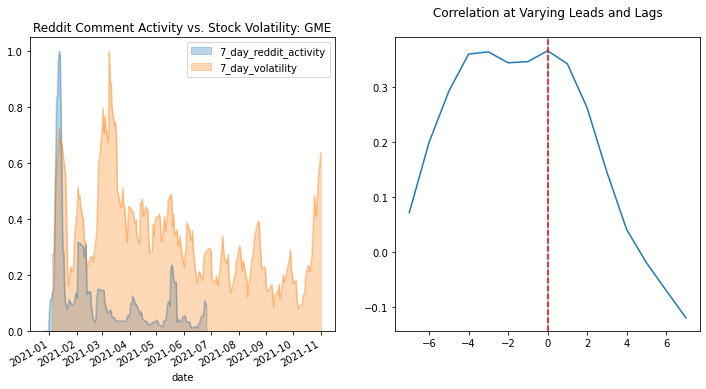In : symbol = 'AMC' lookback = 7 # load, transform, and scale the reddit post data df = read_file(symbol) df['pct_tickers'] = df.apply(lambda row: count_tickers(str(row['title'])+' '+str(row['selftext']), symbol), axis=1) print(f'There were {(df.pct_tickers==0).sum()} false positives to remove...') df = df[df.pct_tickers>0.] # remove false positives entirely df['date'] = pd.to_datetime(df['date_utc'].str[:10]) df['wtd_comments'] = df['num_comments']*df['pct_tickers'] comments = df.groupby('date')['wtd_comments'].sum().rolling(lookback).mean().rename(f'{lookback}_day_reddit_activity') comments = comments.divide(comments.max()) # load and scale the price volatility data path = f'/Users/Chad/data/tiingo/prices/{symbol}.csv' prices = pd.read_csv(path, parse_dates=['date'],index_col='date')['close_adj'] volatility = prices.diff().abs().divide(prices).rolling(lookback).mean()['2021':].rename(f'{lookback}_day_volatility') title = f'Reddit Comment Activity vs. Stock Volatility: {symbol}' volatility =volatility.divide(volatility.max()) # Calculate the lead/lag relationship lag_vals = list(range(-7,8)) corrs = pd.Series() for lag in lag_vals: corr = volatility.shift(lag,freq='d').corr(comments) corrs.loc[lag] = corr max_corr = corrs.max() max_corr_lag = corrs.idxmax(max_corr) # Draw plots fig,axs = plt.subplots(1,2, figsize=(12,6)) # timeseries plot comments.plot(kind='area', ax=axs, alpha = 0.3, legend=True, ylim=(0,None), title=title) volatility.plot(kind='area', ax=axs, alpha = 0.3, legend=True, ylim=(0,None)) # lead/lag plot corrs.plot(title = f'Correlation at Varying Leads and Lags\n',ax=axs) axs.axvline(x=0,color='grey',alpha=0.5) axs.axvline(x=max_corr_lag,ls='--',color='red') ax.set_xlabel("Days Lag between comment volume and price volatility\n (-) means reddit activity LEADS volatility \n (+) means reddit activity LAGS volatility")  <ipython-input-4-434762af2c17>:10: DeprecationWarning: The default dtype for empty Series will be 'object' instead of 'float64' in a future version. Specify a dtype explicitly to silence this warning. s = pd.Series(stocks)  There were 504 false positives to remove...  <ipython-input-13-a432753f57e2>:24: DeprecationWarning: The default dtype for empty Series will be 'object' instead of 'float64' in a future version. Specify a dtype explicitly to silence this warning. corrs = pd.Series()  Out: Text(0.5, 40.400000000000006, 'Days Lag between comment volume and price volatility\n (-) means reddit activity LEADS volatility \n (+) means reddit activity LAGS volatility')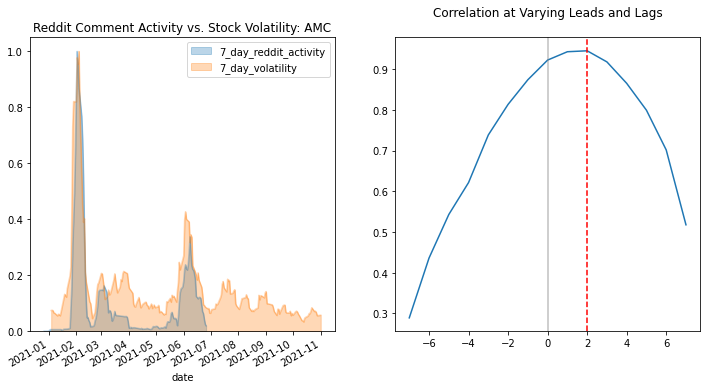Results are inconclusive. $GME shows that reddit leads price, $TSLA falls somewhere between reddit leading and the two values being coincident, and $AMC shows that comment volume may lag price volatility. Unsatisfying to be certain, but such is life in quant research. Any hypotheses or alternative constructions to examine this relationship, please comment below!

## Summary¶

In the third and final post of the series, we will look inside the content of reddit comments, performing some basic sentiment analysis to see if we can discover a more reliable relationship between reddit activity and stock market trend.

## Try it Out!¶

Enough reading, already! The above code is available on colab at the link below. Feel free to try it out yourself.Feel free to modify the notebook if you'd like to experiement. YOu can also "fork" from my notebook (all you'll need is a Google Drive to save a copy of the file...) and extend it to answer your own questions through data.

## One last thing...¶

If you've found this post useful or enlightening, please consider subscribing to the email list to be notified of future posts (email addresses will only be used for this purpose...). To subscribe, scroll to the top of this page and look at the right sidebar.

You can also follow me on twitter (@data2alpha) and forward to a friend or colleague who may find this topic interesting.

In [ ]: